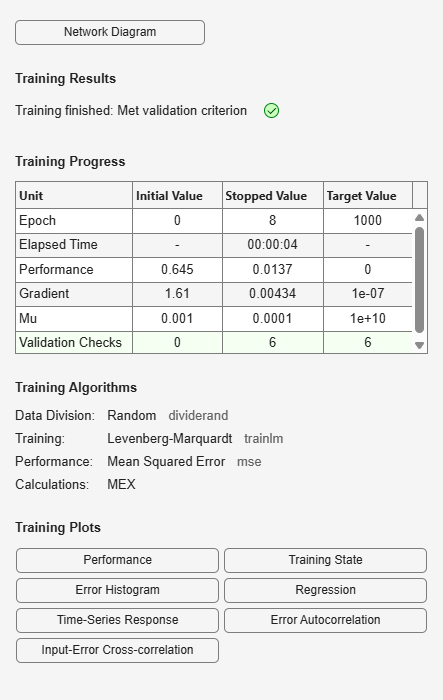# distdelaynet

Distributed delay network

## Syntax

```distdelaynet(delays,hiddenSizes,trainFcn) ```

## Description

Distributed delay networks are similar to feedforward networks, except that each input and layer weights has a tap delay line associated with it. This allows the network to have a finite dynamic response to time series input data. This network is also similar to the time delay neural network (`timedelaynet`), which only has delays on the input weight.

`distdelaynet(delays,hiddenSizes,trainFcn)` takes these arguments,

 `delays` Row vector of increasing 0 or positive delays (default = 1:2) `hiddenSizes` Row vector of one or more hidden layer sizes (default = 10) `trainFcn` Training function (default = `'trainlm'`)

and returns a distributed delay neural network.

## Examples

### Distributed Delay Network

Here a distributed delay neural network is used to solve a simple time series problem.

```[X,T] = simpleseries_dataset; net = distdelaynet({1:2,1:2},10); [Xs,Xi,Ai,Ts] = preparets(net,X,T); net = train(net,Xs,Ts,Xi,Ai); view(net) Y = net(Xs,Xi,Ai); perf = perform(net,Y,Ts) ```
```perf = 0.0323 ```